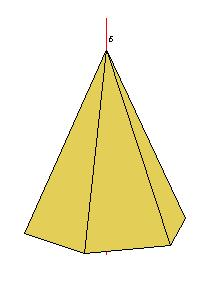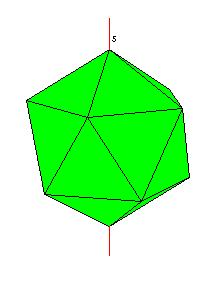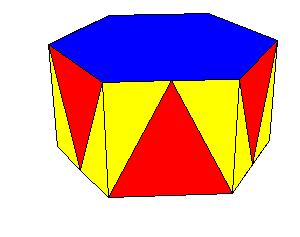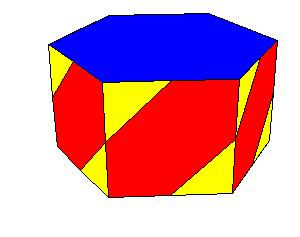# 3D illustrations to Chapter 8 of Cromwell's Polyhedra

#### Izidor Hafner Tomislav Zitko Faculty of Electrical Engineering, University of Ljubljana Trzaska 25 , 1000 Ljubljana , Slovenia e-mail: izidor.hafner@fe.uni-lj.si

One way to improve teaching of stereometry is to give 3D illustrations to well known textbooks. As an example let us take Chapter 8 of Cromwell's Polyhedra. Chapter 8 is dealing with symmetry.

Systems of rotational symmetry Cyclic symmetryFigure 8.1. A rotation axis in a cyclic system

Dihedral symmetryFigure 8.2. Principal and secondary axes in a dihedral systemFigure 8.3. When n is even, the secondary axes in Dn can be separated into two kindsFigure 8.4. Polyhedra with D2 symmetry.

Tetrahedral symmetryFigure 8.5. Rotation axes in the tetrahedral system.

Octahedral symmetryFigure 8.6. Rotation axes in the octahedral system.

Icosahedral SymmetryFigure 8.7. Rotation axes in the icosahedral system.

Reflection symmetryFigure 8.9. A polyhedron with bilateral symmetry.

Prismatic symmetry types

Symmetry type Dnh.Figure 8.11.

Symmetry type Dnv.Figure 8.12.

Symmetry type Dn.Figure 8.13.

Symmetry type CnvFigure 8.14.

Symmetry type CnhFigure 8.15.

Symmetry type Cn.Figure 8.16.

Compound symmetry and the S2n symmetry typeFigure 8.17.reflection in a plane reflection in a point Figure 8.20.

Cubic symmetry types
Symmetry type Oh.Figure 8.21. The reflection planes of a cube.

Symmetry type OFigure 8.22.

Symmetry type Th.Figure 8.23.Figure 8.25.

Symmetry type TFigure 8.26.Figure 2.27.

Some examples

The cube has octahedral rotational symmetry.

The dodecahedron has icosahedral rotational symmetry.

We get examples of tetrahedral symmetry by colouring polyhedra with octahedral and icosahedral symmetry.

References

 P. R. Cromwell, Polyhedra, Cambridge University Press 1997.
 Martin Kraus' Live3D applet